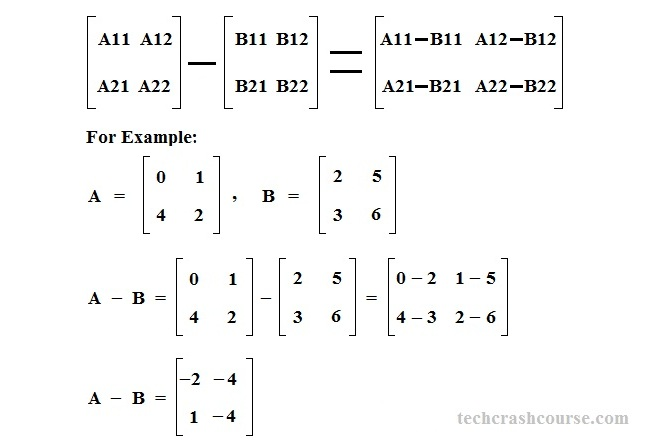# C Program to Subtract of Two Matrices

Here is the C program for subtraction of two matrix. A matrix is a two-dimentional array of elements. The size of a matrix is defined as the number of rows and columns of that matrix.

The horizontal and vertical lines of elements in a matrix are called rows and columns of a matrix. The element in the i-th row and j-th column of a matrix A is referred as (i,j), or A[i,j].
Subtracting two same-size matrices is very similar to adding matrices with the only difference being subtracting corresponding entries.

• Matrix subtraction is defined for two matrices of the same dimensions, If the size of matrices are not same , then the difference of these two matrices is said to be undefined.
• The subtraction of two M × N matrices A and B, denoted by A - B, is again an M × N matrix computed by subtracting corresponding elements.
Algorithm to subtract of two matrices
Let A and B are two matrices of dimension M X N and D is the difference matrix(D = A - B). Below mentioned steps explains how we can find difference of two matrices.
• To subtract two matrices we have to subtract their corresponding elements. For example, D[i][j] = A[i][j] - B[i][j].
• Traverse both matrices row wise(first all elements of a row, then jump to next row) using two loops(check line number 29 and 30 of below program).
• For every element A[i][j], subtract it with corresponding element B[i][j] and store the result in Difference matrix at D[i][j].## C Program for subtraction two matrix

```#include <stdio.h>

int main(){
int rows, cols, rowCounter, colCounter;
int firstMatrix, secondMatrix, differenceMatrix;

printf("Enter Rows and Columns of Matrix\n");
scanf("%d %d", &rows, &cols);

printf("Enter first Matrix of size %dX%d\n", rows, cols);
/*  Input first matrix*/
for(rowCounter = 0; rowCounter < rows; rowCounter++){
for(colCounter = 0; colCounter < cols; colCounter++){
scanf("%d", &firstMatrix[rowCounter][colCounter]);
}
}
/*  Input second matrix*/
printf("Enter second Matrix of size %dX%d\n", rows, cols);
for(rowCounter = 0; rowCounter < rows; rowCounter++){
for(colCounter = 0; colCounter < cols; colCounter++){
scanf("%d", &secondMatrix[rowCounter][colCounter]);
}
}
/* Subtract corresponding elements of both matrices
differenceMatrix[i][j] = firstmatrix[i][j] + secondMatrix[i][j] */
for(rowCounter = 0; rowCounter < rows; rowCounter++){
for(colCounter = 0; colCounter < cols; colCounter++){
differenceMatrix[rowCounter][colCounter] = firstMatrix[rowCounter][colCounter]
- secondMatrix[rowCounter][colCounter];
}
}
/* Print difference matrix*/
printf("Difference Matrix\n");
for(rowCounter = 0; rowCounter < rows; rowCounter++){
for(colCounter = 0; colCounter < cols; colCounter++){
printf("%d ", differenceMatrix[rowCounter][colCounter]);
}
printf("\n");
}

return 0;
}
```
Output
```Enter Rows and Columns of Matrix
2 2
Enter first Matrix of size 2X2
0 2
1 3
Enter second Matrix of size 2X2
3 2
1 0
Difference Matrix
-3 0
0 3
```
```Enter Rows and Columns of Matrix
3 2
Enter first Matrix of size 3X2
2 1
0 1
7 2
Enter second Matrix of size 3X2
0 0
-2 1
2 -3
Difference Matrix
2 1
2 0
5 5
```

Related Topics
 C program to add two matrix C Program to print a matrix diagonally C Program to find sum of diagonal elements of matrix C Program for matrix multiplication C program to print upper triangular matrix C program to compare two matrix C Program to print fibonacci series C Program to calculate factorial of a number List of all C programs